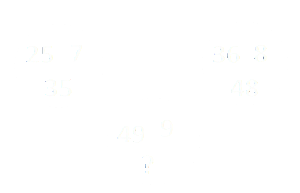Home > Math Shortcuts > Brain Trigger #39 – Sample Number puzzle question

# Brain Trigger #39 – Sample Number puzzle question

Now here we will discuss few question answers on Number puzzle which are very common in competitive exams. These Number puzzle practice question answer session will help you to prepare for your examination. Try to solve the question mention below using your skills on math. You can use shortcut methods to solve these Number puzzle questions.

We try every types of Number puzzle questions and their shortcut methods to bring together here in this website. Now you just need to apply those tricks to solve these questions. However it’s possible that you may not need any tricks to solve few questions.

Here we will discuss a question on Number puzzle. What you need to do is to, read the question carefully and then try yourself to solve the question. Answer will be provided below. If you do this problem then check the solution of this question with your answer. If you don’t know how to do it then check the solution below.

Each page is having a question on Number puzzle and also contain its solution with detail explanation. By cliking on Next/Previous link you can navigate through other questions.

Let’s begin the Question answer session. Today’s question is:

What shouldv be the number replaces in the question mark ?Answer of this question is provided below. Scroll down to see the answer. Take as much time as you want to get the answer of this question. But always try to do it as faster as you can.

(Scroll down to get the answer)

(Scroll down to get the answer)

(Scroll down to get the answer)

(Scroll down to get the answer)

Here is the Answer of above question. If you already done this by yourself then please check your answer with us. Well Done if you got an correct answer of the question, but if you are not able to answer it correctly then don’t worry. Here is the solution of the problem with explanation.

You can solve in this way……….

5 square = 25, 6 square = 36, 7 square = 49
then,
5 x 7 = 35
6 x 8 = 48
7 x 9 = 63